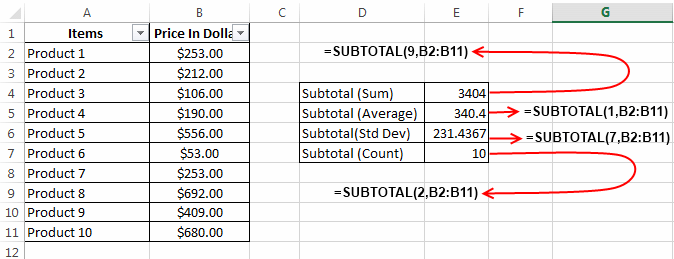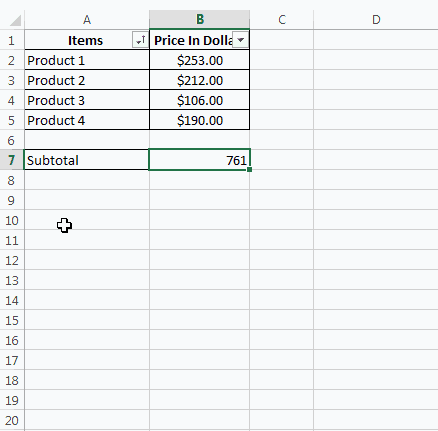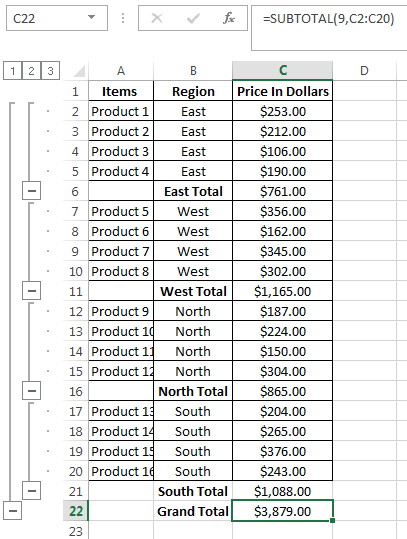# SubTotal Function In Excel – How to Use

Subtotal Function in Excel is a wonderful formula that can be used to perform some specific arithmetic and logical operation on a defined range of cells.

Microsoft Excel defines Subtotal Function as “It returns a subtotal in a list or database”.

Subtotal formula takes two arguments:
1. Operation Code
2. Range of Cells.

In the below section we will see the syntax of Subtotal.

### Syntax of Subtotal Function:

Subtotal Function can be written as:

`=subtotal(Operation_Code, Range1)`

Here ‘`Operation_Code`’ specifies the type of mathematical operation that you like to perform on the specified range of cells.

Operation CodeOperation NameDescription
1AVERAGECalculates the Average of specified range.
2COUNTCounts the number of cells in a specified range.
3COUNTACounts the number of non-empty cells in a specified range.
4MAXFinds the Largest Value in the specified range.
5MINFinds the Smallest Value in the specified range.
6PRODUCTCalculates the product of cells in specified range.
7STDEVEstimates Standard Deviation in the specified range.
8STDEVPCalculates Standard Deviation based on the entire population.
9SUMCalculates the Sum of specified range.
10VAREstimates variance in the specified range.
11VARPEstimates variance based on the entire population.

`Range1`’ signifies the array of cells.

### Example of Subtotal Function in Excel:

In the below example I have used multiple subtotal functions.The formula `=SUBTOTAL(9,B2:B11)` results into the Sum (operation code = 9) of all the elements present in the range B2:B11.

And `=SUBTOTAL(1,B2:B11)` results into the Average (operation code = 1) of all the elements present in the range B2:B11

In the same way `=SUBTOTAL(7,B2:B11)` results into the Standard Deviation (operation code = 7) of specified the range.

### Why you should use Subtotal function?

I know after reading about subtotal function you will have a question in your mind, why should I use Subtotal function to perform any mathematical operation if I have a separate function already available for this.

For instance: You know that with subtotal you can do sum of a specified range but excel has already given you sum function for this. So why should you use Subtotal?

The answer to the above question is simple. Subtotal gives you two important capabilities over traditional functions.

1. It can produce results dynamically: If you try to use excel Subtotal function on a particular range, then you will see that its results are generated dynamically. You can understand it clearly by seeing the below example.In the above example the subtotal value changes dynamically according to the filter.

2. Feature that ignores hidden rows: You must be knowing that excel has a feature ‘Hide rows’. Many times when you have some irrelevant records in your data you hide them. But if you apply traditional sum function over this range, then you won’t be able to exclude these hidden records. But Subtotal Function gives you this ability i.e. you can only count or sum the visible records in a range.

But this needs you to use special operational codes in the subtotal function. And you get these special operational codes by adding 100 to the original ones. For example the average operational code is 1 so 101 will be the operational code for average function which excludes hidden rows.

Example:

`=subtotal(9,B2:B5)` will give you sum of all the values (including hidden) between B2 and B5.

And `=subtotal(109,B2:B5)` will give you sum of all visible values (excluding hidden) between B2 and B5.

### Using Subtotal Function from Excel Ribbon:

You can also use the subtotal function from the Excel ribbon, ‘Data’ tab > ‘Subtotal’ option. Using the formula from this option is quite easy. Using subtotal formula from this option creates collapse and expand options (+/-) on the left side of the rows. This makes it easier for you to view your data without scrolling.To use this Subtotal option simply follow the below steps:

1. Select your table, and Navigate to Data > Subtotal.2. This will open a subtotal window. In the option ‘At each change in’ select the column based on which you want to consolidate the result (for my example I have used the column ‘Region’). Next select the appropriate function to be used and finally check the column to which you wish to apply the formula.

3. Click the ‘Ok’ button and the formula will be applied.About Ankit Kaul

Ankit is the founder of Excel Trick. He is tech Geek who loves to sit in front of his square headed girlfriend (his PC) all day long. :D. Ankit has a strong passion for learning Microsoft Excel. His only aim is to turn you guys into 'Excel Geeks'.

1.MMsays

How can I reduce data using the Subtotal range method and copy it to a new range at the same time?

eg col1 col2
A 1
A 2
B 2

if the above data is a particular range, i need the output in another range and the result should be

col1 col2
A 3
B 2

Thanks,

•Ankit Kaulsays

Hi MM,

2.Jaztin A.says

•Ankit Kaulsays